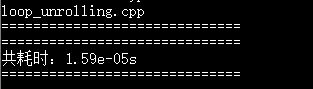# C++性能剖析教程之循环展开

更新时间：2018年06月16日 08:50:43   作者：Root lee

• 减少了不直接有助于程序结果的操作的数量，例如循环索引计算和分支条件。
• 提供了一些方法，可以进一步变化代码，减少整个计算中关键路径上的操作数量。

```#include <iostream>
#include <chrono>

int main(){
auto start = std::chrono::system_clock::now();
int sum = 0;
int count = 10000;
//循环10000次累加
for(int i = 0;i < count;i++){
sum += i;
}
auto end = std::chrono::system_clock::now();
std::chrono::duration<double> dura = end - start;
std::cout <<"共耗时："<< dura.count() << "s" << std::endl;
return 0;
}``````for(int i = 0;i < count;i += 2){
sum += i;
sum += i+1;
}``````int sum1=0,sum2=0;
for(int i=0;i < count;i+=2){
sum1 += i;
sum2 += i+1;
}
sum = sum1 + sum2;```